Abhishek Sharma — April 14, 2020

The world is governed by chance. Randomness stalks us every day of our lives.

– Paul Auster

Random numbers are all around us in the world of data science. Every so often I need to quickly draw up some random numbers to run a thought experiment, or to demonstrate a concept to an audience but without having to download big datasets.

From creating dummy data to shuffling the data for training and testing purposes or initializing weights of a neural network, we generate random numbers all the time in Python. You’ll love this concept once you have the hang of it after this article.In my opinion, generating random numbers is a must-know topic for anyone in data science. I’ll guide you through the entire random number generation process in Python here and also demonstrate it using different techniques.

New to Python? These two free courses will get you started:

• Random Library
• Seeding Random Numbers
• Generating Random Numbers in a Range
• uniform()
• randint()
• Picking Up Randomly From a List
• Shuffling a List
• Generating Random Numbers According to Distributions
• gauss()
• expovariate()

## Generating Random Numbers in Python using the Random Library

Here’s the good news – there are various ways of generating random numbers in Python. The easiest method is using the random module. It is a built-in module in Python and requires no installation. This module uses a pseudo-random number generator (PRNG) known as Mersenne Twister for generating random numbers.

A pseudo-random number generator is a deterministic random number generator. It does not generate truly random numbers. It takes a number as an input and generates a random number for it.

Note: Do not use the random module for generating random numbers for security purposes. For security and cryptographic uses, you can use the secrets module which uses true-random number generators (TRNG).

## Seeding Random Numbers

As we discussed in the above section, the random module takes a number as input and generates a random number for it. This initial value is known as a seed, and the procedure is known as seeding.

The numbers we generate through pseudo-random number generators are deterministic. This means they can be replicated using the same seed.

Let’s understand it with an example:

```import random

print('Random Number 1=>',random.random())
print('Random Number 2=>',random.random())```

Here, I am using the random() function which generates a random number in the range [0.0, 1.0]. Notice here that I haven’t mentioned the value of the seed. By default, the current system time in milliseconds is used as a seed. Let’s take a look at the output.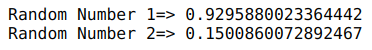Both numbers are different because of the change in time during execution from the first statement to the second statement. Let’s see what happens if we seed the generators with the same value:

```random.seed(42)
print('Random Number 1=>',random.random())

random.seed(42)
print('Random Number 2=>',random.random())```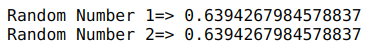We get the same numbers here. That’s why pseudo-random number generators are deterministic and not used in security purposes because anyone with the seed can generate the same random number.

## Generating Random Numbers in a Range

So far, we know about creating random numbers in the range [0.0, 1.0]. But what if we have to create a number in a range other than this?

One way is to multiply and add numbers to the number returned by the random() function. For example, random.random() * 3 + 2 will return numbers in the range [2.0, 5.0]. However, this is more of a workaround, not a straight solution.

Don’t worry! The random module has got your back here. It provides uniform() and randint() functions that we can use for this purpose. Let’s understand them one by one.

### uniform()

The uniform() function of the random module takes starting and ending values of a range as arguments and returns a floating-point random number in the range [starting, ending]:

`print('Random Number in range(2,8)=>', random.uniform(2,8))`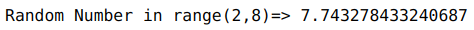### randint()

This function is similar to the uniform() function. The only difference is that the uniform() function returns floating-point random numbers, and the randint() function returns an integer. It also returns the number in the range [starting, ending]:

`print('Random Number in a range(2,8)=>', random.randint(2,8))`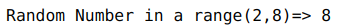## Picking Up Randomly From a List

choice() & choices() are the two functions provided by the random module that we can use for randomly selecting values from a list. Both of these functions take a list as an argument and randomly select a value(s) from it. Can you guess what the difference between choice() and choices() is?

choice() only picks a single value from a list whereas choices() picks multiple values from a list with replacement. One fantastic thing about these functions is that they work on a list containing strings too. Let’s see them in action:

```a=[5, 9, 20, 10, 2, 8]
print('Randomly picked number=>',random.choice(a))
print('Randomly picked number=>',random.choices(a,k=3))```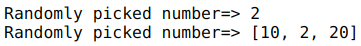As you can see, choice() returned a single value from a and choices() returned three values from a. Here, k is the length of the list returned by choices().

One more thing you can notice in the responses returned by choices() is that each value occurs only once. You can increase the probability of each value being picked by passing an array as weights to the choices() function. So, let’s increase the probability of 10 to as much as thrice of others and see the results:

```for _ in range(5):
print('Randomly picked number=>',random.choices(a,weights=[1,1,1,3,1,1],k=3))```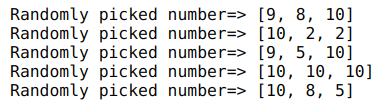Here, we can see that 10 occurred in every draw from the list. There also exists a sample() function in the random module that works similarly to the choices() function but takes random samples from a list without replacement.

## Shuffling a List

Let’s say we don’t want to pick values from a list but you just want to reorder them. We can do this using the shuffle() function from the random module. This shuffle() function takes the list as an argument and shuffles the list in-place:

```print('Original list=>',a)
random.shuffle(a)
print('Shuffled list=>',a)```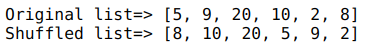Note: The shuffle() function does not return a list.

## Generating Random Numbers According to Distributions

One more amazing feature of the random module is that it allows us to generate random numbers based on different probability distributions. There are various functions like gauss(), expovariate(), etc. which help us in doing this.

If you are not familiar with probability distributions, then I highly recommend you to read this article: 6 Common Probability Distributions every data science professional should know.

### gauss()

Let’s start with the most common probability distribution, i.e., normal distribution. gauss() is a function of the random module used for generating random numbers according to a normal distribution. It takes mean and standard deviation as an argument and returns a random number:

```for _ in range(5):
print(random.gauss(0,1))```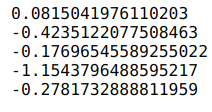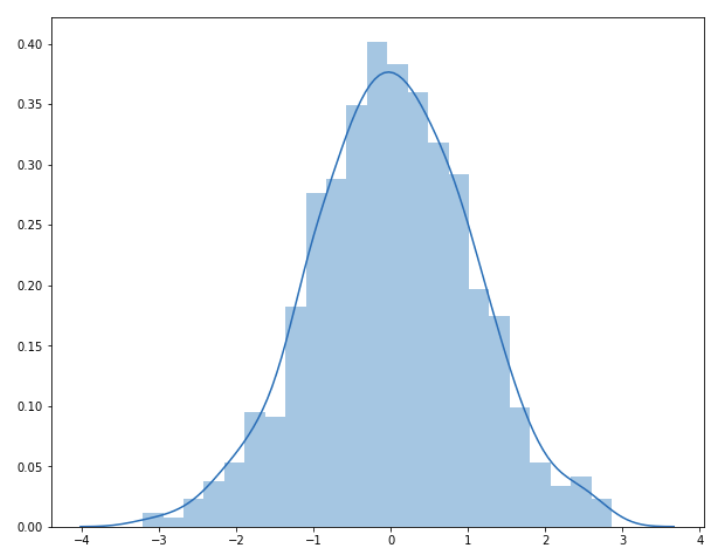Here, I plotted 1000 random numbers generated by the gauss() function for mean equal to 0 and standard deviation as 1. You can see above that all the points are spread around the mean and they are not widely spread since the standard deviation is 1.

### expovariate()

Exponential distribution is another very common probability distribution that you’ll encounter. The expovariate() function is used for getting a random number according to the exponential distribution. It takes the value of lambda as an argument and returns a value from 0 to positive infinity if lambda is positive, and from negative infinity to 0 if lambda is negative:

`print('Random number from exponential distribution=>',random.expovariate(10))`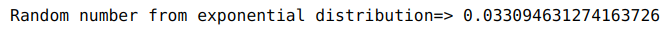## End Notes

I often use random numbers for creating dummy datasets and for random sampling. I’d love to know how you use random numbers in your projects so comment down below with your thoughts and share them with the community.

If you found this article informative, then please share it with your friends and comment below your queries and feedback. I have listed some amazing articles related to Python and data science below for your reference: